# Covariance functions

Covariance functions (also called kernels) are the key ingredient in using Gaussian processes. They encode all assumptions about the form of function that we are modelling. In general, covariance represents some form of distance or similarity. Consider two input points (locations) $$x_i$$ and $$x_j$$ with corresponding observed values $$y_i$$ and $$y_j$$. If the inputs $$x_i$$ and $$x_j$$ are close to each other, we generally expect that $$y_i$$ and $$y_j$$ will be close as well. This measure of similarity is embedded in the covariance function.

The Ariadne library currently implements only the squared exponential covariance function.

## Squared exponential kernel

$k(x_i, x_j) = \sigma^2 \exp\left( - \frac{(x_i - x_j)^2}{2 l^2} \right) + \delta_{ij} \sigma_{\text{noise}}^2$

where $$\sigma^2 > 0$$ is the signal variance, $$l > 0$$ is the lengthscale and $$\sigma^2_{\text{noise}} >= 0$$ is the noise covariance. The noise variance is applied only when $$i = j$$.

Squared exponential is appropriate for modelling very smooth functions. The parameters have the following interpretation:

• Lengthscale $$l$$ describes how smooth a function is. Small lengthscale value means that function values can change quickly, large values characterize functions that change only slowly. Lengthscale also determines how far we can reliably extrapolate from the training data.

Small lengthscale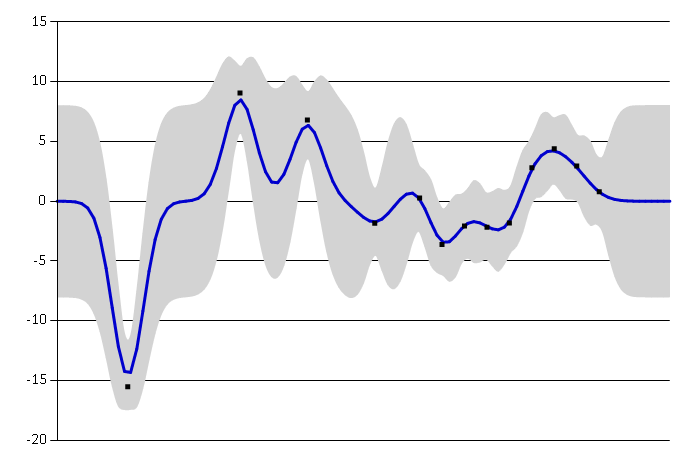Large lengthscale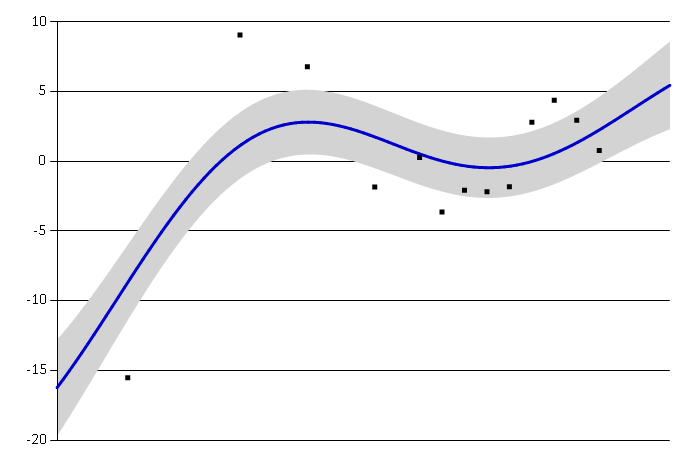• Signal variance $$\sigma^2$$ is a scaling factor. It determines variation of function values from their mean. Small value of $$\sigma^2$$ characterize functions that stay close to their mean value, larger values allow more variation. If the signal variance is too large, the modelled function will be free to chase outliers.

Small signal variance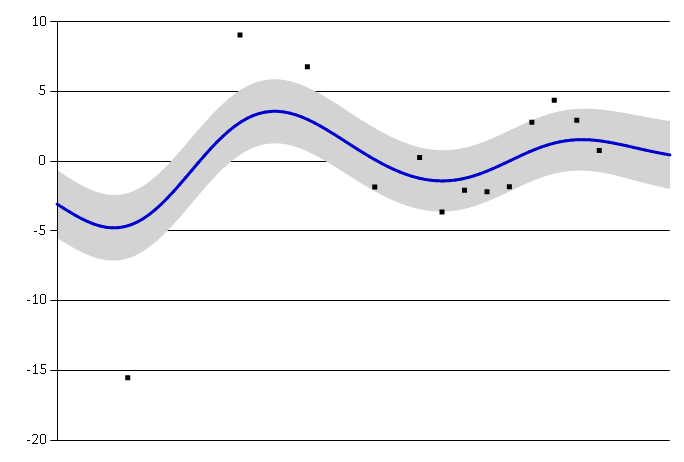Large signal variance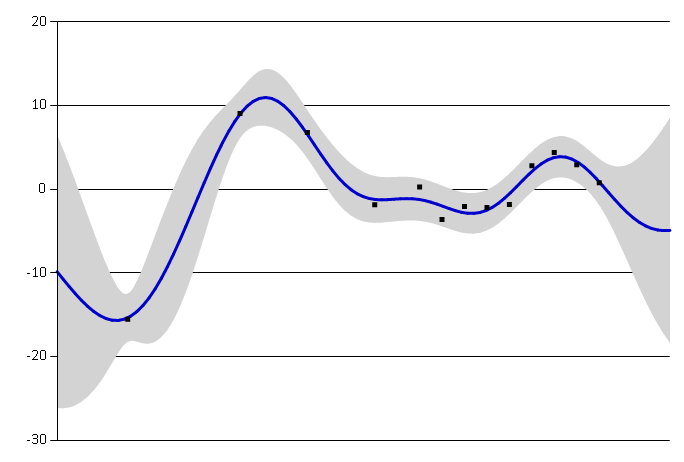• Noise variance $$\sigma^2_{\text{noise}}$$ is formally not a part of the covariance function itself. It is used by the Gaussian process model to allow for noise present in training data. This parameter specifies how much noise is expected to be present in the data.

Small noise variance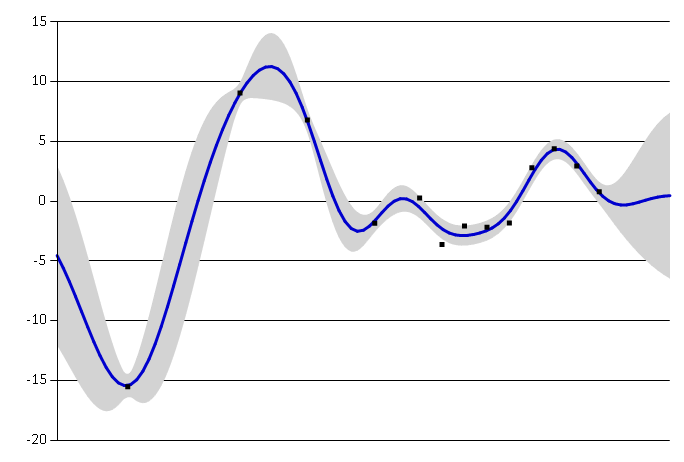Large noise variance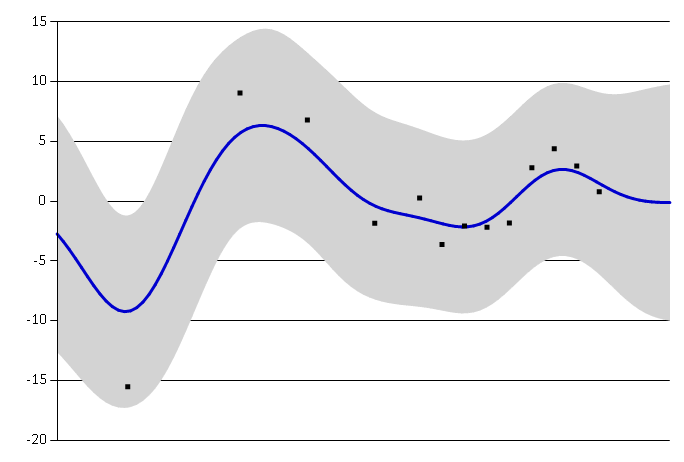Squared exponential kernel can be created from its parameters.

 1: 2: 3: 4: 5: 6: 7: 8:  open Ariadne.Kernels // hyperparameters let lengthscale = 3.0 let signalVariance = 15.0 let noiseVariance = 1.0 let sqExp = SquaredExp.SquaredExp(lengthscale, signalVariance, noiseVariance)
Squared exponential, l = 3.00, σ² = 15.00, σ²_{noise} = 1.00


We can also use it to directly initialize a Gaussian process.

 1:  let gp = sqExp.GaussianProcess()

Information on how to select parameters for the squared exponential automatically are in the Optimization section of this website.

## Creating custom covariance functions

Any covariance function can be used in conjunction with Gaussian processes in Ariadne. Gaussian process constructor requires simply a function which takes a pair of input locations and computes their covariance.

General covariance function (kernel) has the following type:

 1:  type Kernel<'T> = 'T * 'T -> float

For example, we can define a simple linear kernel as follows:

 1: 2: 3: 4: 5: 6: 7: 8: 9:  open Ariadne.GaussianProcess let linearKernel (x1, x2) = let var = 1.0 let bias = 0.0 let offset = 0.0 bias + var * (x1 - offset)*(x2 - offset) let gpLinear = GaussianProcess(linearKernel, Some 1.0)

This covariance function corresponds to a non-efficient way of doing Bayesian linear regression.

module Kernels

val lengthscale : float

Full name: CovarianceFunctions.lengthscale
val signalVariance : float

Full name: CovarianceFunctions.signalVariance
val noiseVariance : float

Full name: CovarianceFunctions.noiseVariance
val sqExp : SquaredExp.SquaredExp

Full name: CovarianceFunctions.sqExp
module SquaredExp

Full name: CovarianceFunctions.gp
member SquaredExp.SquaredExp.GaussianProcess : unit -> Ariadne.GaussianProcess.GaussianProcess<float>
type Kernel<'T> = 'T * 'T -> float

Full name: covarianceFunctions.Kernel<_>
Multiple items
val float : value:'T -> float (requires member op_Explicit)

Full name: Microsoft.FSharp.Core.Operators.float

--------------------
type float = System.Double

Full name: Microsoft.FSharp.Core.float

--------------------
type float<'Measure> = float

Full name: Microsoft.FSharp.Core.float<_>
module GaussianProcess

val linearKernel : x1:float * x2:float -> float

Full name: CovarianceFunctions.linearKernel
val x1 : float
val x2 : float
val var : float
val bias : float
val offset : float
val gpLinear : GaussianProcess<float>

Full name: CovarianceFunctions.gpLinear
Multiple items

--------------------
new : covariance:Kernel<'T> * noiseVariance:float option -> GaussianProcess<'T>
new : covariance:Kernel<'T> * meanFunction:MeanFunction * noiseVariance:float option -> GaussianProcess<'T>
union case Option.Some: Value: 'T -> Option<'T>